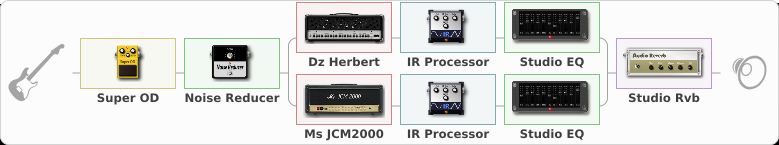# Fear Factory Industrial

Discussion in 'ToneLib-GFX presets' started by Tetragramm1983, Nov 24, 2020.

1. ### Tetragramm1983Active Member

Fear Factory Industrial

Preset name: Industrial Metal Preset

For that Industrial Metal Thickness. Comparible to Fear Factory

Effects chain:Effect: "Super OD" (Overdrive / Distortion), active - "yes"
{
"Drive" = 65
"Tone" = 70
"Level" = 100
}

Effect: "Noise Reducer" (Dynamics / Filter), active - "yes"
{
"Sens" = 81
"Mode" = Hard
}

Effect: "Splitter" (Dynamics / Filter), active - "yes"
{
"A-Bypass" = Off
"A-Pan" = 0
"A-Level" = 55
"B-Bypass" = Off
"B-Pan" = 0
"B-Level" = 55

'A' branch:
{

Effect: "Dz Herbert" (Amp simulators), active - "yes"
{
"Gain" = 35
"Bass" = 65
"Middle" = 35
"Treble" = 80
"Presence" = 75
"Master" = 70
"Level (dB)" = 2
}

Effect: "IR Processor" (Cabinets), active - "yes"
{
"IR" = ACE EV 5153ST 1a
"Low Cut (Hz)" = 58
"Hi Cut (kHz)" = 20.0
"Mix" = 100
"Level (dB)" = 1
}

Effect: "Studio EQ" (Dynamics / Filter), active - "yes"
{
"31 Hz" = -2
"62 Hz" = -2
"125 Hz" = 4
"250 Hz" = -2
"500 Hz" = -4
"1 kHz" = 4
"2 kHz" = 4
"4 kHz" = 4
"8 kHz" = 4
"16 kHz" = 1
"Level (dB)" = 0
}
}
'B' branch:
{

Effect: "Ms JCM2000" (Amp simulators), active - "yes"
{
"Gain" = 35
"Bass" = 65
"Middle" = 25
"Treble" = 80
"Presence" = 85
"Master" = 70
"Level (dB)" = 2
}

Effect: "IR Processor" (Cabinets), active - "yes"
{
"IR" = ACE EV 5153ST 4b
"Low Cut (Hz)" = 41
"Hi Cut (kHz)" = 20.0
"Mix" = 100
"Level (dB)" = 2
}

Effect: "Studio EQ" (Dynamics / Filter), active - "yes"
{
"31 Hz" = -2
"62 Hz" = -2
"125 Hz" = 4
"250 Hz" = -2
"500 Hz" = -4
"1 kHz" = 4
"2 kHz" = 4
"4 kHz" = 4
"8 kHz" = 4
"16 kHz" = 1
"Level (dB)" = 0
}
}
}

Effect: "Studio Rvb" (Reverberation), active - "yes"
{
"Time" = 7.3
"PreDelay" = 48
"LoDamp" = 21
"HiDamp" = 23
"Mix" = 30
}

Note: You will need to download and install the ToneLib-GFX software to use the preset.

#### Attached Files:

• ###### Fear_Factory_Industrial.tlgfx
File size:
14.9 KB
Views:
2,389
Ars570, jem and HelIlcat like this.